# Solution to Grand Quiz Question #2

From figure we have,

 PR = QR
 QS = SR
-----------------------------------------------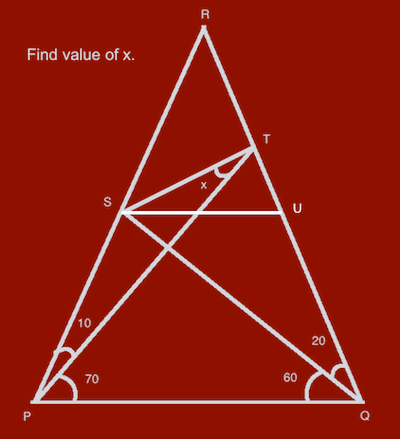In Figure 2, parallel to PQ, we draw SU
Because  and PQ || SU, we get
 RS =RU
 PS = QU
-----------------------------------------------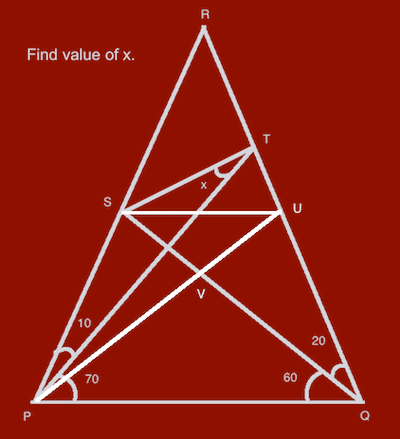In Figure 3, we draw line PU.
From , and PQ = QP, and angle RPQ = RQP (=80),
the triangle PQS equals to triangle PQU.
So
 PU = QS
From , PU = SR
From , we get
 PU = RU
So angle RPU = 20, TPU = 10, UPQ = 60,
 SVU = 60
 PQ = QV = VP
From ,  and , we get
 SU = UV = VS
-----------------------------------------------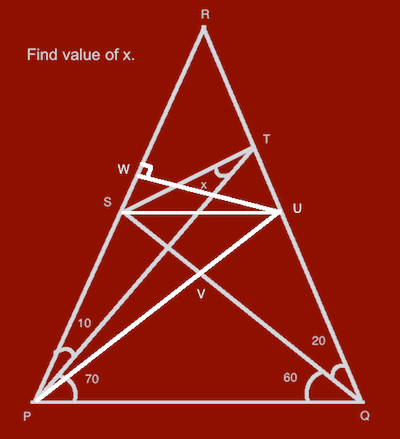In Figure 4, we draw UW vertical to PR.
From  and angle RPU = PRU, we get triangle PUW equals to triangle RUW. So
 PW = RW
-----------------------------------------------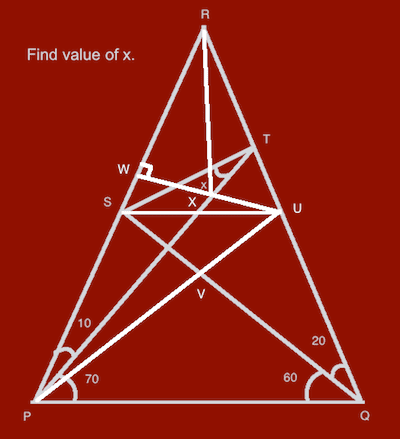Figure 5
In Figure 5, we draw RX.
From  and UW vertical to PR, we get
 angle SRX = SPX = 10
 RX = PX
From , we get
 angle URX = 10 = SRX
[13'] angle URX = UPX

## Replies

•Ankita Katdare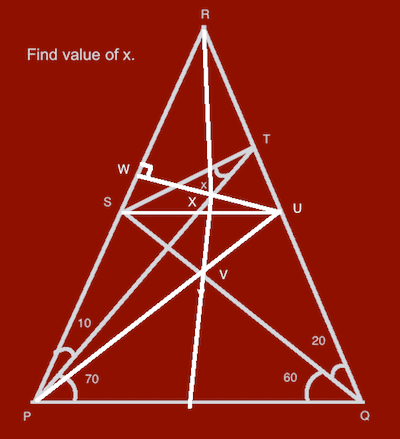Figure 6

In Figure 6, extend RX to PQ.
From , and angle PQR = QPR = 80, we get
line RX vertical to PQ,
 line RX is the same as line RV
From , , , we get triangle RTX equals to triangle PVX. So
 TX = VX
From , , we get
 RV = PT
From [13'], , we get triangle RVU equals to triangle PTU. So
 TU = UV
--------------------------------------------------------

Finally
From , , we get
 TU = SU
Because SU || PQ, so
 angle RUS = RQP = 80
From , , we get
 angle STU = TSU = 50
So angle x = angle STU - angle PTU
= 50 - (180 - 70 - 60 - 20)
= 50 - 30
= 20
--------------------------------------------------------

This is just one of the solutions.
The fun about geometry is that, the same problem can have different approaches to land up to a solution.

We are expecting some logical approach (may be) different than what I mentioned above. 😀
•Ankita Katdare
Yesterday's question's Coins will not be credited to anyone.

But the solution finding is still open to all.
Please come up with better solutions.

😀
•ISHAN TOPRE
Yesterday's question's Coins will be credited to Issue. 😀
By the way here is the new solution.
If you are allowed to use a compass, actually drawing the figure will give you answer in 30 Sec. Do try it. Its fun.
All you need to do is to draw an Isosceles triangle with any suitable base length.
Remember that we have to play with angles. The length is immaterial. I did it. 😀
•PraveenKumar Purushothaman
Woah... Didn't expect it goes this much... Anyways, my solution was from Hand Drawing and CorelDraw. 😀

You are reading an archived discussion.

## When I will be requiring Wireless Router?

Consider a scenario, where I have one BroadBand Connection from BSNL with a "WiFi enabled option" modem. So we can access internet through our phone (to a certain limit, which...

## power electronics

how to make use of the cmos transistor for power electronics applications??? if anyone knows any applications... please reply... 😔

## Grand Quiz Question #3

The 3rd Question of the CE Grand Quiz Contest is as follows - Q. Answer the question and fill in the blanks based on the following image. [Question is worth...

## Downtimes & Errors On CrazyEngineers

CEans, I apologize for the frequent downtime and errors you might have experienced in the last two days. I along with our webhost admins are fighting a database related problem...

## Transient response of a transmission line

I want to know dat what is meant by transient response of a transmission line. I have searched different sites and i am unable to find what i want.. my...